# Closure space

(diff) ← Older revision | Latest revision (diff) | Newer revision → (diff)

Letbe a set and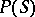the set of subsets of. A function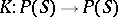is a closure operation if for all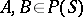:

K1)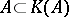;

K2)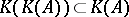;

K3)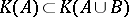;

K4)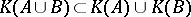;

K5)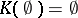. These are the Kuratowski closure axioms, and a functionsatisfying these axioms is called a Kuratowski closure operator (or Kuratowski closure operation).

A pair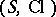is a closure space if and only if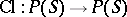satisfies: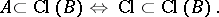This condition is equivalent to K1)–K3). A closure space that satisfies K5) is a Fréchet-space. A Fréchet-space that satisfies K4) is a topological space.

A Čech closure operator is a mapping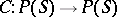such that

C1);

C2) (K1))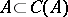;

C3)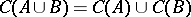. A set endowed with a Čech closure operator is called a pre-topological space.

How to Cite This Entry:
Closure space. Encyclopedia of Mathematics. URL: http://encyclopediaofmath.org/index.php?title=Closure_space&oldid=13257
This article was adapted from an original article by M. Hazewinkel (originator), which appeared in Encyclopedia of Mathematics - ISBN 1402006098. See original article